Select Page

So far, in our ML.NET journey, we were focused on computer vision problems like Image Classification and Object Detection. In this article, we change a direction a bit and explore NLP (Natural Language Processing) and the set of problems we can solve with machine learning.

Natural language processing (NLP) is a subfield of artificial intelligence with the main goal to help programs understand and process natural language data. The output of this process is a computer program that can “understand” language.

Are you afraid that AI might take your job? Make sure you are the one who is building it.

STAY RELEVANT IN THE RISING AI INDUSTRY! 🖖

Back in 2018, Google presented a paper with a deep neural network called Bidirectional Encoder Representations from Transformers or BERT. Because of its simplicity, it became one of the most popular NLP algorithms. With this algorithm, anyone can train their own state-of-the-art question answering system (or a variety of other models) in just a few hours. In this article, we will do just that, use BERT to create a question and answering system.BERT is a neural network that is based on Transformers architecture. That is why in this article we will first explore that architecture a bit and then continue to a more advanced understanding of BERT:

## 1. Prerequisites

The implementations provided here are done in C#, and we use the latest .NET 5. So make sure that you have installed this SDK. If you are using Visual Studio this comes with version 16.8.3. Also, make sure that you have installed the following packages:

``````\$ dotnet add package Microsoft.ML

You can do the same from the Package Manager Console:

``````Install-Package Microsoft.ML
Install-Package Microsoft.ML.OnnxRuntime
Install-Package Microsoft.ML.OnnxTransformer``````

You can do a similar thing using Visual Studio’s Manage NuGetPackage option: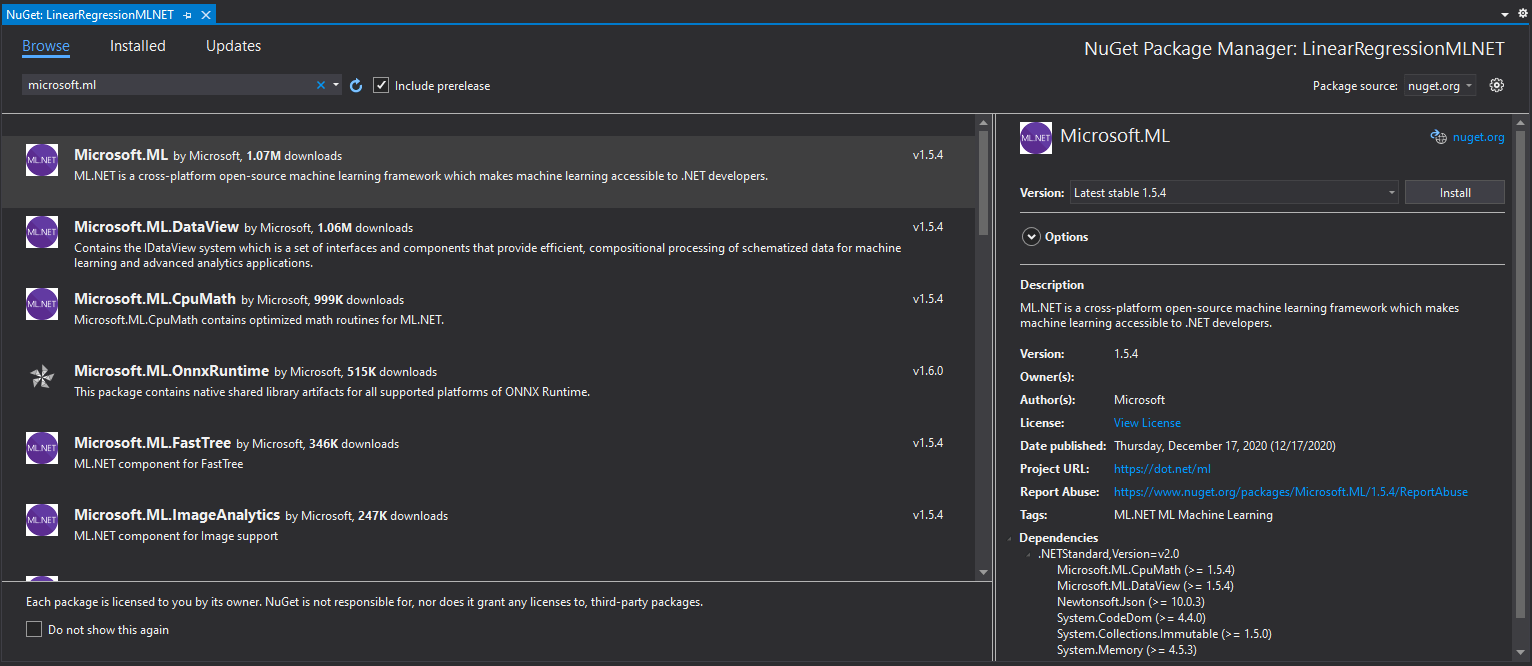If you need to catch up with the basics of machine learning with ML.NET check out this article.

## 2. Understanding Transformers Architecture

Language is sequential data. Basically, you can observe it as a stream of words, where the meaning of each word is depending on the words that came before it and from the words that come after it. That is why computers have such a hard time understanding language because in order to understand one word you need a context.

Also, sometimes as the output, you need to provide a sequence of data (words) as well. A good example to demonstrate this is the translation of English into Serbian. As an input to the algorithm, we use a sequence of words and for the output, we need to provide a sequence as well.

In this example, an algorithm needs to understand English and to understand how to map  English words to Serbian words (inherently this means that some understanding of Serbian must exist as well). Over the years there were many deep learning architectures used for this purpose., like Recurrent Neural Networks and LSTMs. However, it was the use of Transformer architecture that changed everything.RNNs and LSTMs networks didn’t fully satisfy the need, since they are hard to train and prone to vanishing (and exploding) gradient. Transformers aimed to solve these problems and bring better performance and a better understanding of the language. They were introduced back in 2017. in the legendary paper Attention is all you need

In a nutshell, they used Encoder-Decoder structure and self-attention layers to better understand language. If we go back to the translation example, the Encoder is in charge of understanding English, while the Decoder is in charge of understanding Serbian and mapping English to Serbian.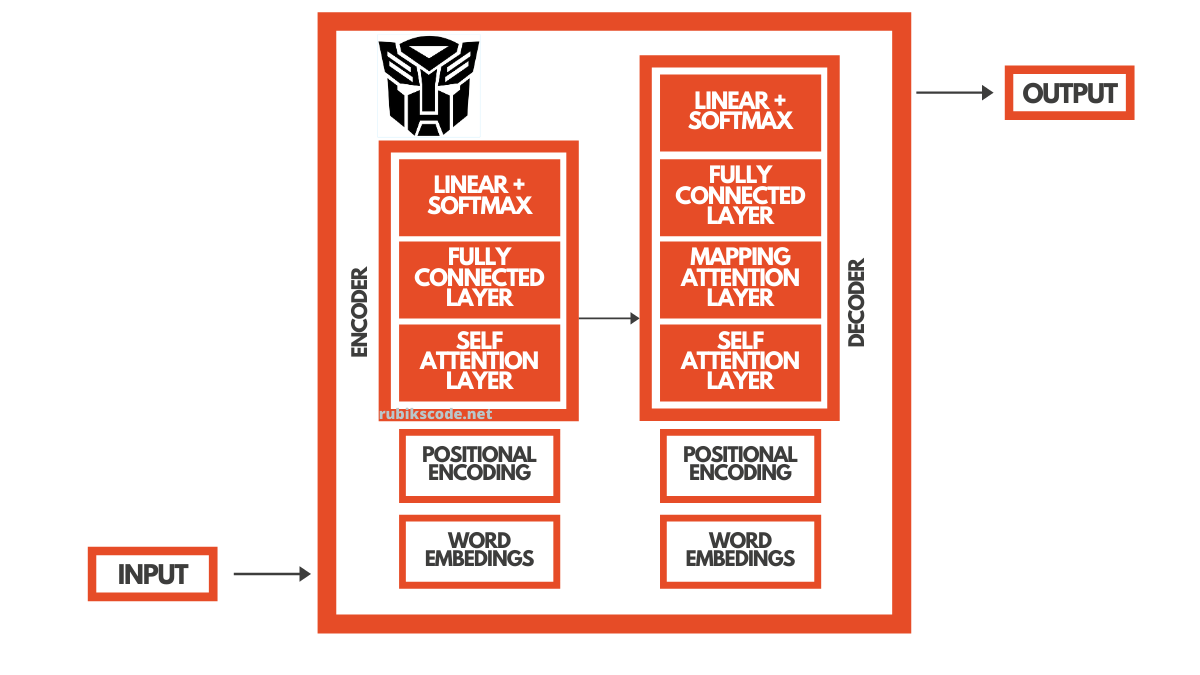During the training, process Encoder is supplied with word embeddings from the English language. Computers don’t understand words, they understand numbers and matrixes (set of numbers). That is why we convert words into some vector space, meaning we assign certain vectors (map them to some latent vector space) to each word in the language. These are word embeddings. There are many available word embeddings like Word2Vec.

However, the position of the word in the sentence is also important for the context. That is why positional encoding is done. That is how the encoder gets information about the word and its context. The Self-attention layer of the Encoder is determining the relation between the words and gives us information about how relevant each word of the sentence is. This is how Encoder understands English. Data then goes to the deep neural network and then to the Mapping-Attention Layer of the Decoder.

However, before that, the Decoder gets the same information about the Serbian language. It learns how to understand the Serbian language, in the same way, using word embeddings, positional encoding and self-attention. Mapping-Attention Layer of the Decoder then has both information, about the English language and about Serbian language and it just learns how to words from one language to another. To learn more about Transformers, check out this article.

## 3. BERT Intuition

BERT used this Transformers architecture to understand language. To be more precise it utilizes Encoder. This architecture achieved two big milestones. First, it achieved bidirectionality. This means that every sentence is learned in both ways, and context is better learned, both previous context and future context. BERT is the first deeply bidirectionalunsupervised language representation, pre-trained using only a plain text corpus (Wikipedia). It is also one of the first pre-trained models for NLP. We learned about transfer learning for computer vision. However, before BERT this concept didn’t really pick up in the world of NLP.

This makes a lot of sense because you can train a model on a lot of data and once it understands the language, you can fine-tune it for more specific tasks. That is why the training of BERT can be separated into two phases: Pre-training and Fine Tuning.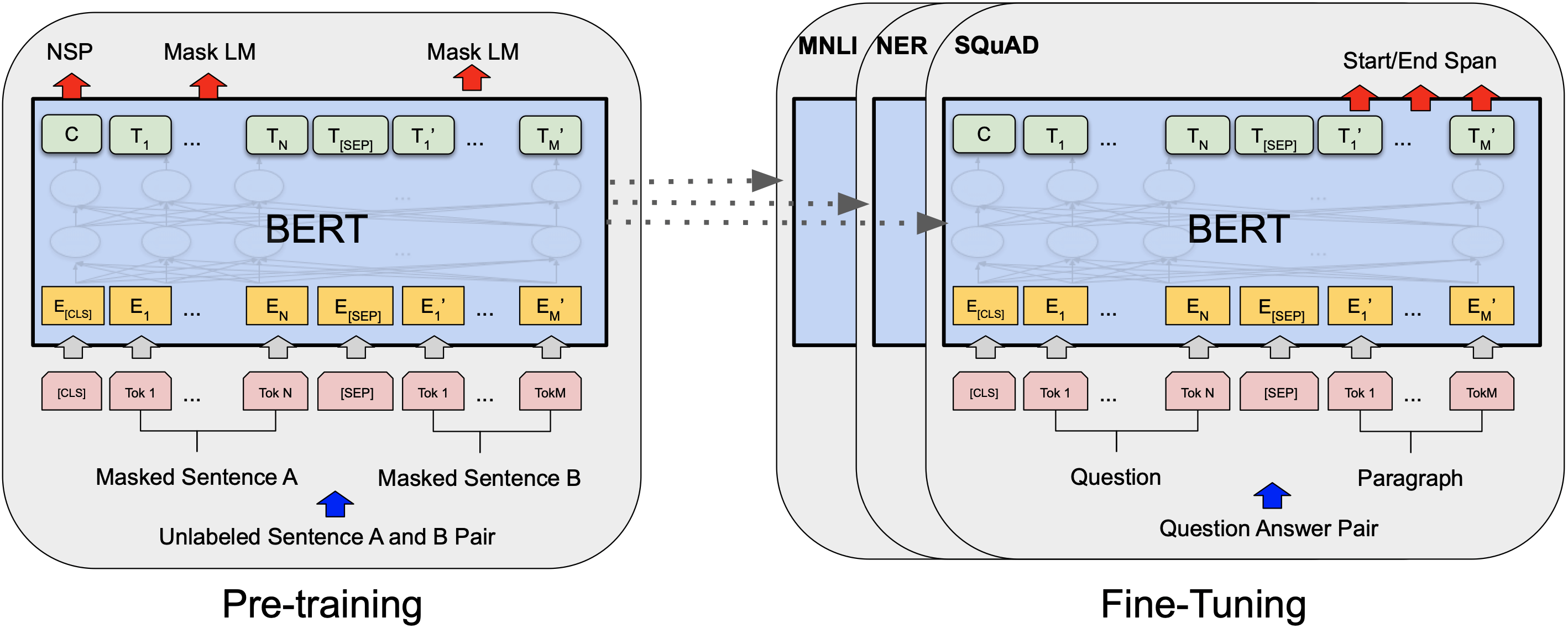In order to achieve bidirectionality BERT is pre-trained with two methods:

• Masked Language Modeling – MLM
• Next Sentence Prediction – NSP

The Masked Language Modeling uses masked input. This means that some words in the sentence are masked and it is BERT’s job to fill in the blanks. Next Sentence Prediction is giving two sentences as an input and expects from BERT to predict is one sentence following another. In reality, both of these methods happen at the same time.During the fine tuning phase we train BERT for specific task. This means that if we want to create question answering solution, we need to train just additional layers of BERT. This is exactly what we do in this tutorial. All we need to do is to replace output layers of the network, with the fresh set of layers designed for our specific purpose. As an input we will have a passage of the text (or context) and a question, and as the output we expect the answer to the question.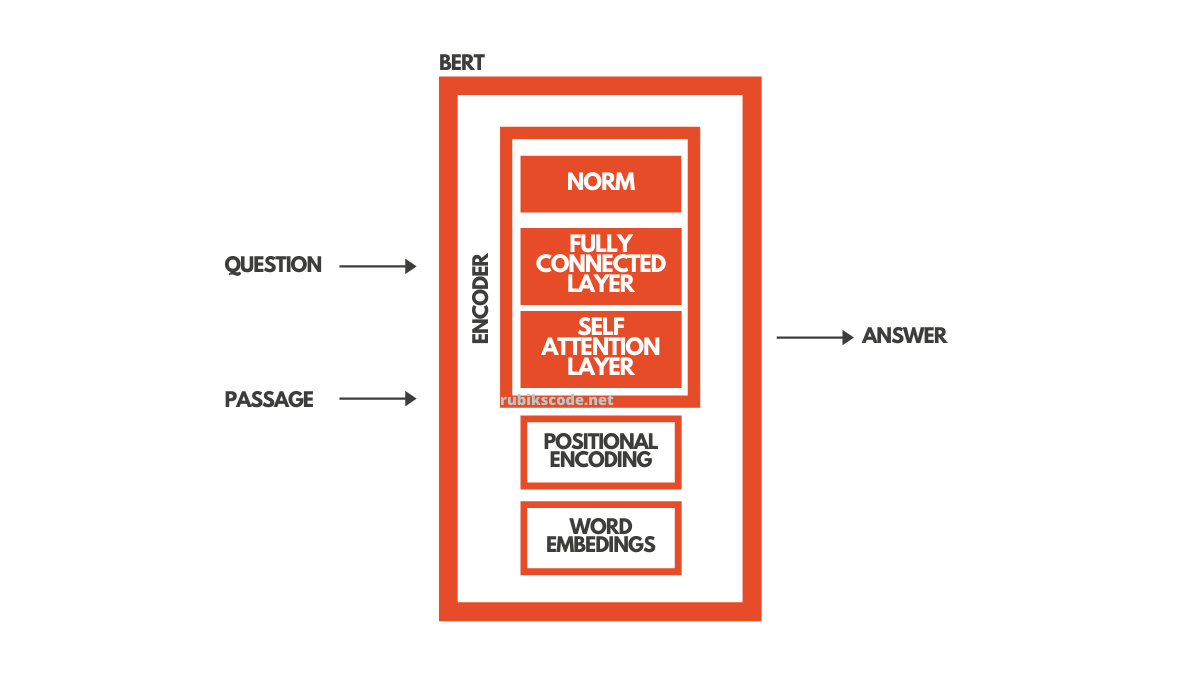For example, our system that should use two sentences: “Jim is walking through the woods.” (passage or context) and “What is his name?” (question) to provide the answer “Jim”.

## 4. ONNX Models

Before we dive into the implementation of object detection application with ML.NET we need to cover one more theoretical thing. That is the Open Neural Network Exchange (ONNX) file format. This file format is an open-source format for AI models and it supports interoperability between frameworks.

Basically, you can train a model in one machine learning framework like PyTorch, save it and convert it into ONNX format. Then you can consume that ONNX model in a different framework like ML.NET. That is exactly what we do in this tutorial. You can find more information on the ONNX website.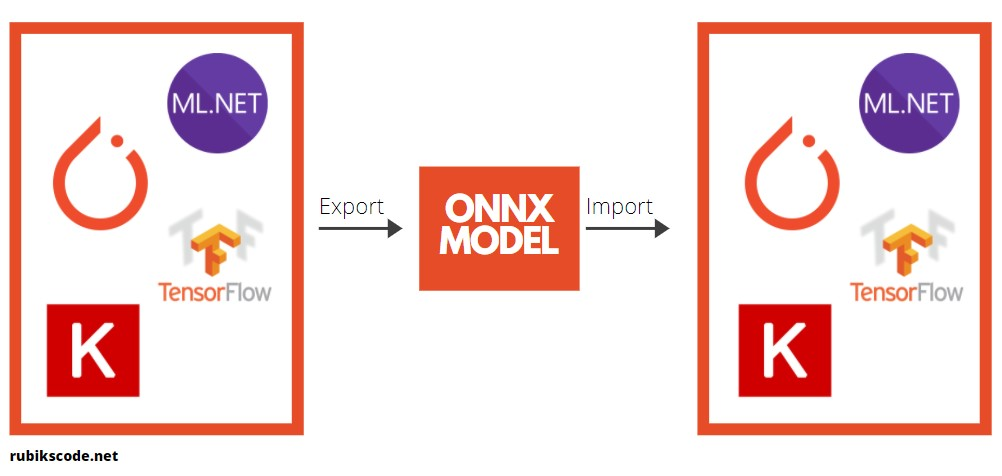In this tutorial, we use the pre-trained BERT model. This model is available here a the BERT SQUAD. In essence, we import this model into ML.NET and run it within our application.

One very interesting and useful thing we can do with the ONNX model is that there are a bunch of tools we can use for a visual representation of the model. This is very useful when we use pre-trained models as we do in this tutorial.

We often need to know the names of input and output layers, and this kind of tool is good for that. So, once we download the BERT model, we can load it with one of the tools for visual representation. In this guide, we use Netron and here is just the part of the output:I know, it insane, BERT is a big model. You might wonder how can I use this and why do I need it? However, in order to work with ONNX models, we usually need to know the names of input and output layers of the model. Here is how that looks for BERT: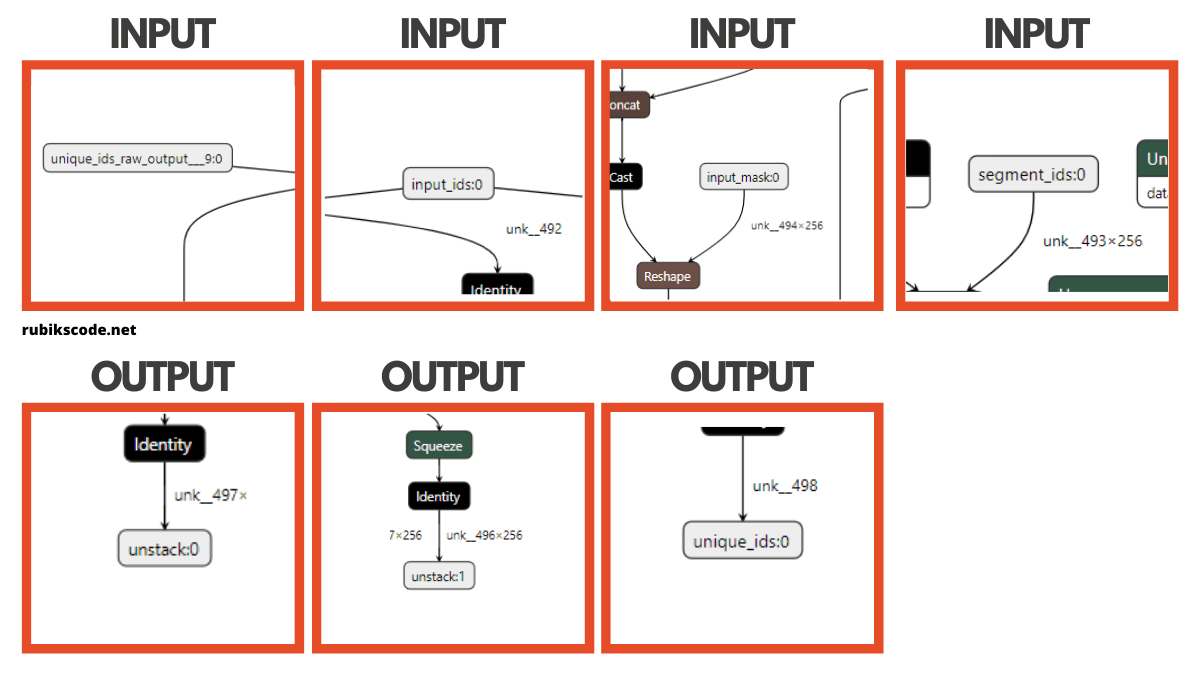## 5. Implementation with ML.NET

If you take a look at the BERT-Squad repository from which we have downloaded the model, you will notice somethin interesting in the dependancy section. To be more precise, you will notice dependancy of tokenization.py. This means that we need to perform tokenization on our own. Word tokenization is the process of splitting a large sample of text into words. This is a requirement in natural language processing tasks where each word needs to be captured and subjected to further analysis. There are many ways to do this.

Effectively, we perform word encoding and for that we use Word-Piece Tokenization as explained in this paper. It is ported version from the tokenzaton.py. To implement this complute solution we structured ur solution like this: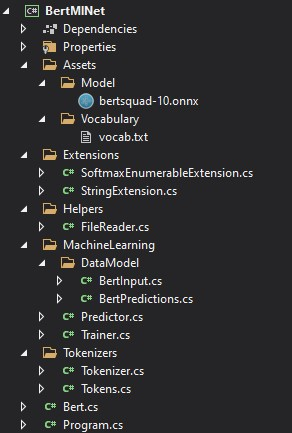Here in the Assets folder, you can find the downloaded .onnx model and folder with vocabulary on which we want to train our model. The Machine Learning folder contains all the necessary code that we use in this application. The Trainer and Predictor classes are there, just like the classes which are modeling data. In the separate folder, we can find helper class for loading files and extension classes for Softmax on Enumerable type and splitting of the string.This solution is inspired by the implementation of Gjeran Vlot which can be found here.

### 5.1 Data Models

You may notice that in the DataModel folder we have two classes, for input and predictions of BERT. The BertInput class is there to represent the input. They are names and sized like the layers from the model:

``````using Microsoft.ML.Data;

namespace BertMlNet.MachineLearning.DataModel
{
public class BertInput
{
[VectorType(1)]
[ColumnName("unique_ids_raw_output___9:0")]
public long[] UniqueIds { get; set; }

[VectorType(1, 256)]
[ColumnName("segment_ids:0")]
public long[] SegmentIds { get; set; }

[VectorType(1, 256)]
public long[] InputMask { get; set; }

[VectorType(1, 256)]
[ColumnName("input_ids:0")]
public long[] InputIds { get; set; }
}
}
``````

The Bertpredictions class uses BERT output layers:

``````using Microsoft.ML.Data;

namespace BertMlNet.MachineLearning.DataModel
{
public class BertPredictions
{
[VectorType(1, 256)]
[ColumnName("unstack:1")]
public float[] EndLogits { get; set; }

[VectorType(1, 256)]
[ColumnName("unstack:0")]
public float[] StartLogits { get; set; }

[VectorType(1)]
[ColumnName("unique_ids:0")]
public long[] UniqueIds { get; set; }
}
}
``````

### 5.2 Trainer

The Trainer class is quite simple, it has only one method BuildAndTrain which uses the path to the pre-trained model.

``````using BertMlNet.MachineLearning.DataModel;
using Microsoft.ML;
using System.Collections.Generic;

namespace BertMlNet.MachineLearning
{
public class Trainer
{

public Trainer()
{
_mlContext = new MLContext(11);
}

public ITransformer BuidAndTrain(string bertModelPath, bool useGpu)
{
var pipeline = _mlContext.Transforms
.ApplyOnnxModel(modelFile: bertModelPath,
outputColumnNames: new[] { "unstack:1",
"unstack:0",
"unique_ids:0" },
inputColumnNames: new[] {"unique_ids_raw_output___9:0",
"segment_ids:0",
"input_ids:0" },
gpuDeviceId: useGpu ? 0 : (int?)null);

}
}
}
``````

In the mentioned method, we build the pipeline. Here we apply the ONNX model and connect data models to the layers of the BERT ONNX model. Note that we have a flag that we can use to train this model on CPU or on GPU. Finally, we fit this model to empty data. We do this, so we can load the data schema, ie. to load the model.

### 5.3 Predictor

The Predictor class is even more simple. It receives a trained and loaded model and creates a prediction engine. Then it uses this prediction engine to create predictions on new images.

``````using BertMlNet.MachineLearning.DataModel;
using Microsoft.ML;

namespace BertMlNet.MachineLearning
{
public class Predictor
{
private MLContext _mLContext;
private PredictionEngine<BertInput, BertPredictions> _predictionEngine;

public Predictor(ITransformer trainedModel)
{
_mLContext = new MLContext();
_predictionEngine = _mLContext.Model
.CreatePredictionEngine<BertInput, BertPredictions>(trainedModel);
}

public BertPredictions Predict(BertInput encodedInput)
{
return _predictionEngine.Predict(encodedInput);
}
}
}
``````

### 5.4 Helpers and Extensions

There is one helper class and two extension classes. The helper class FileReader has a method for reading text file. We use it later to load vocabulary from file. It is very simple:

``````using System.Collections.Generic;
using System.IO;

namespace BertMlNet.Helpers
{
{
{
var result = new List<string>();

{
string line;

{
if (!string.IsNullOrWhiteSpace(line))
{
}
}
}

return result;
}
}
}
``````

There are two extension classes. One for performing Softmax operation on collection of elements and another one for spliting the string and yealding one result at a time.

``````using System;
using System.Collections.Generic;
using System.Linq;

namespace BertMlNet.Extensions
{
public static class SoftmaxEnumerableExtension
{
public static IEnumerable<(T Item, float Probability)> Softmax<T>(
this IEnumerable<T> collection,
Func<T, float> scoreSelector)
{
var maxScore = collection.Max(scoreSelector);
var sum = collection.Sum(r => Math.Exp(scoreSelector(r) - maxScore));

return collection.Select(r => (r, (float)(Math.Exp(scoreSelector(r) - maxScore) / sum)));
}
}
}
``````
``````using System.Collections.Generic;

namespace BertMlNet.Extensions
{
static class StringExtension
{
public static IEnumerable<string> SplitAndKeep(
this string inputString, params char[] delimiters)
{
int start = 0, index;

while ((index = inputString.IndexOfAny(delimiters, start)) != -1)
{
if (index - start > 0)
yield return inputString.Substring(start, index - start);

yield return inputString.Substring(index, 1);

start = index + 1;
}

if (start < inputString.Length)
{
yield return inputString.Substring(start);
}
}
}
}
``````

### 5.4 Tokenizer

Ok, so far we explored simple parts of the soluton. Let’s proceed with the more complicated and important ones, let’s check out how we implemted tokenization. First, we define the list of default BERT tokens. For example, two sentences should always be separated with the token `[SEP]` to differentiate them. The `[CLS]` token always appears at the start of the text, and is specific to classification tasks.

``````namespace BertMlNet.Tokenizers
{
public class Tokens
{
public const string Padding = "";
public const string Unknown = "[UNK]";
public const string Classification = "[CLS]";
public const string Separation = "[SEP]";
}
}
``````

The process of Tokenization is done within Tokenizer class. There are two public methods: Tokenize and Untokenize. The first one first splits received text into sentences. Then for each sentence each word is transformed into embedding. Note it can happen that one word is represened with multiple tokens.

For example, word “embeddings” is represented as array of tokens `['em', '##bed', '##ding', '##s']`. The word has been split into smaller subwords and characters. The two hash signs preceding some of these subwords are just our tokenizer’s way to denote that this subword or character is part of a larger word and preceded by another subword.

So, for example, the ‘##bed’ token is separate from the ‘bed’ token. Another thing that Tokenize method is doing is returning Vocabulary Index and Segmentation Index. Both are used as an BERT input. To learn more why is this done this way, check out this article.

``````using BertMlNet.Extensions;
using System;
using System.Collections.Generic;
using System.Linq;

namespace BertMlNet.Tokenizers
{
public class Tokenizer
{

public Tokenizer(List<string> vocabulary)
{
_vocabulary = vocabulary;
}

public List<(string Token, int VocabularyIndex, long SegmentIndex)> Tokenize(params string[] texts)
{
IEnumerable<string> tokens = new string[] { Tokens.Classification };

foreach (var text in texts)
{
tokens = tokens.Concat(TokenizeSentence(text));
tokens = tokens.Concat(new string[] { Tokens.Separation });
}

var tokenAndIndex = tokens
.SelectMany(TokenizeSubwords)
.ToList();

var segmentIndexes = SegmentIndex(tokenAndIndex);

=> (tokenindex.Token, tokenindex.VocabularyIndex, segmentindex)).ToList();
}

public List<string> Untokenize(List<string> tokens)
{
var currentToken = string.Empty;
var untokens = new List<string>();
tokens.Reverse();

tokens.ForEach(token =>
{
if (token.StartsWith("##"))
{
currentToken = token.Replace("##", "") + currentToken;
}
else
{
currentToken = token + currentToken;
currentToken = string.Empty;
}
});

untokens.Reverse();

return untokens;
}

public IEnumerable<long> SegmentIndex(List<(string token, int index)> tokens)
{
var segmentIndex = 0;
var segmentIndexes = new List<long>();

foreach (var (token, index) in tokens)
{

if (token == Tokens.Separation)
{
segmentIndex++;
}
}

return segmentIndexes;
}

private IEnumerable<(string Token, int VocabularyIndex)> TokenizeSubwords(string word)
{
if (_vocabulary.Contains(word))
{
return new (string, int)[] { (word, _vocabulary.IndexOf(word)) };
}

var tokens = new List<(string, int)>();
var remaining = word;

while (!string.IsNullOrEmpty(remaining) && remaining.Length > 2)
{
var prefix = _vocabulary.Where(remaining.StartsWith)
.OrderByDescending(o => o.Count())
.FirstOrDefault();

if (prefix == null)
{

}

remaining = remaining.Replace(prefix, "##");

}

if (!string.IsNullOrWhiteSpace(word) && !tokens.Any())
{
}

}

private IEnumerable<string> TokenizeSentence(string text)
{
// remove spaces and split the , . : ; etc..
return text.Split(new string[] { " ", "   ", "\r\n" }, StringSplitOptions.None)
.SelectMany(o => o.SplitAndKeep(".,;:\\/?!#\$%()=+-*\"'–_`<>&^@{}[]|~'".ToArray()))
.Select(o => o.ToLower());
}
}
}
``````

Another public method is Untokenize. This method is used to reverse the process. Basically, as the output of BERT we will get varous embeddings. The goal of this method is to convert this information into meaningfull sentences.

This class has multiple methods that are enabeling this process.

### 5.5 BERT

The Bert class puts all these things together. In the constructor, we read the vocabulary file and instantiate Train, Tokenizer and Predictor objects. There is only one public method – Predict. This method receives context and the question. As the output, the answer with the probability is retrieved:

``````using BertMlNet.Extensions;
using BertMlNet.Helpers;
using BertMlNet.MachineLearning;
using BertMlNet.MachineLearning.DataModel;
using BertMlNet.Tokenizers;
using System.Collections.Generic;
using System.Linq;

namespace BertMlNet
{
public class Bert
{
private List<string> _vocabulary;

private Predictor _predictor;

public Bert(string vocabularyFilePath, string bertModelPath)
{
_tokenizer = new Tokenizer(_vocabulary);

var trainer = new Trainer();
var trainedModel = trainer.BuidAndTrain(bertModelPath, false);
_predictor = new Predictor(trainedModel);
}

public (List<string> tokens, float probability) Predict(string context, string question)
{
var tokens = _tokenizer.Tokenize(question, context);
var input = BuildInput(tokens);

var predictions = _predictor.Predict(input);

var contextStart = tokens.FindIndex(o => o.Token == Tokens.Separation);

var (startIndex, endIndex, probability) = GetBestPrediction(predictions, contextStart, 20, 30);

var predictedTokens = input.InputIds
.Skip(startIndex)
.Take(endIndex + 1 - startIndex)
.Select(o => _vocabulary[(int)o])
.ToList();

var connectedTokens = _tokenizer.Untokenize(predictedTokens);

return (connectedTokens, probability);
}

private BertInput BuildInput(List<(string Token, int Index, long SegmentIndex)> tokens)
{
var padding = Enumerable.Repeat(0L, 256 - tokens.Count).ToList();

var tokenIndexes = tokens.Select(token => (long)token.Index).Concat(padding).ToArray();
var segmentIndexes = tokens.Select(token => token.SegmentIndex).Concat(padding).ToArray();

return new BertInput()
{
InputIds = tokenIndexes,
SegmentIds = segmentIndexes,
UniqueIds = new long[] { 0 }
};
}

private (int StartIndex, int EndIndex, float Probability) GetBestPrediction(BertPredictions result, int minIndex, int topN, int maxLength)
{
var bestStartLogits = result.StartLogits
.Select((logit, index) => (Logit: logit, Index: index))
.OrderByDescending(o => o.Logit)
.Take(topN);

var bestEndLogits = result.EndLogits
.Select((logit, index) => (Logit: logit, Index: index))
.OrderByDescending(o => o.Logit)
.Take(topN);

var bestResultsWithScore = bestStartLogits
.SelectMany(startLogit =>
bestEndLogits
.Select(endLogit =>
(
StartLogit: startLogit.Index,
EndLogit: endLogit.Index,
Score: startLogit.Logit + endLogit.Logit
)
)
)
.Where(entry => !(entry.EndLogit < entry.StartLogit || entry.EndLogit - entry.StartLogit > maxLength || entry.StartLogit == 0 && entry.EndLogit == 0 || entry.StartLogit < minIndex))
.Take(topN);

var (item, probability) = bestResultsWithScore
.Softmax(o => o.Score)
.OrderByDescending(o => o.Probability)
.FirstOrDefault();

return (StartIndex: item.StartLogit, EndIndex: item.EndLogit, probability);
}
}
}
``````

The Predict method performs several steps. Let’s explore it in more details.

``````public (List<string> tokens, float probability) Predict(string context, string question)
{
var tokens = _tokenizer.Tokenize(question, context);
var input = BuildInput(tokens);

var predictions = _predictor.Predict(input);

var contextStart = tokens.FindIndex(o => o.Token == Tokens.Separation);

var (startIndex, endIndex, probability) = GetBestPrediction(predictions,
contextStart,
20,
30);

var predictedTokens = input.InputIds
.Skip(startIndex)
.Take(endIndex + 1 - startIndex)
.Select(o => _vocabulary[(int)o])
.ToList();

var connectedTokens = _tokenizer.Untokenize(predictedTokens);

return (connectedTokens, probability);
}``````

First, this method performs tokenization of the question and passed context (passage based on which BERT should give answer). Then we build BertInput from this information. This is done in BertInput method. Basically, all the tokenized information is padded so it can be used as an BERT input and used to initialize BertInput object.

Then we get the predictions of the model from Predictor. This information is then additionaly processed and best predictions from the context is found. Meaning, BERT picks word from the context that are most likely the answer and we pick the best ones. Finally, these words are untokenized.

### 5.5 Program

Program is utilizing what we implemented in the Bert class. First, let’s define launch settings:

``````{
"profiles": {
"BERT.Console": {
"commandName": "Project",
"commandLineArgs": "\"Jim is walking through the woods.\" \"What is his name?\""
}
}
}
``````

We define two command line arguments: “Jim is walking throught the woods.” and “What is his name?”. As we already mentioned, the first one is context and the second one is question. The Main method is minimal:

``````using System;
using System.Text.Json;

namespace BertMlNet
{
class Program
{
static void Main(string[] args)
{
var model = new Bert("..\\BertMlNet\\Assets\\Vocabulary\\vocab.txt",

var (tokens, probability) = model.Predict(args, args);

Console.WriteLine(JsonSerializer.Serialize(new
{
Probability = probability,
Tokens = tokens
}));
}
}
}
``````

Technically we create Bert object with the paths to vocabulary file and path to the model. Then we call Predict method with comand line arguments. As the output we get this:

``{"Probability":0.9111285,"Tokens":["jim"]}``

We can see that BERT is 91% sure that the answer to the question is ‘Jim’ and it is correct.

## Conclusion

In this article, we learned how BERT works. To be more specific, we had a chance to explore different how Transformers architecture works and how BERT utilizes that architecture to understand language. Finally, we learned about ONNX model format and how we can use it with ML.NET.#### Nikola M. Zivkovic

CAIO at Rubik's Code

Nikola M. Zivkovic a CAIO at Rubik’s Code and the author of book “Deep Learning for Programmers“. He is loves knowledge sharing, and he is experienced speaker. You can find him speaking at meetups, conferences and as a guest lecturer at the University of Novi Sad.

Rubik’s Code is a boutique data science and software service company with more than 10 years of experience in Machine Learning, Artificial Intelligence & Software development. Check out the services we provide.

Ultimate Guide to Machine Learning with Python

Everything from Python basics to the deployment of Machine Learning algorithms to production in one place.

Become a Machine Learning Superhero TODAY!